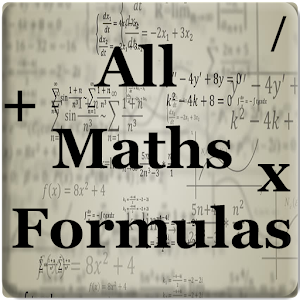# All Maths Formulas - Lumos Educational App Store4.31

#### DESCRIPTION:

Here is maths formulas pack for all android users. Now no need to make paper notes to remember mathematics formulas just have this app put all the formulas on your favorite phones. you'll find formulas very simply explained in app with necessary figures will help you to understand very easily. includes 1. Algebra - Factoring formulas - Product formulas - Roots formula - Powers formula - Logarithmic formula - Useful equations - Complex number - Binomial theorem 2. Geometry - Cone - Cylinder - Isosceles Triangle - Square - Sphere - Rectangle - Rhombus - Parallelogra

#### OVERVIEW:

All Maths Formulas is a free educational mobile app By wasim jaffer.It helps students in grades 8 practice the following standards .

This page not only allows students and teachers download All Maths Formulas but also find engaging Sample Questions, Videos, Pins, Worksheets, Books related to the following topics.

8

Developer: wasim jaffer

Software Version: 1.1

Category: Education

### RELATED APPS:EdSearch WebSearch